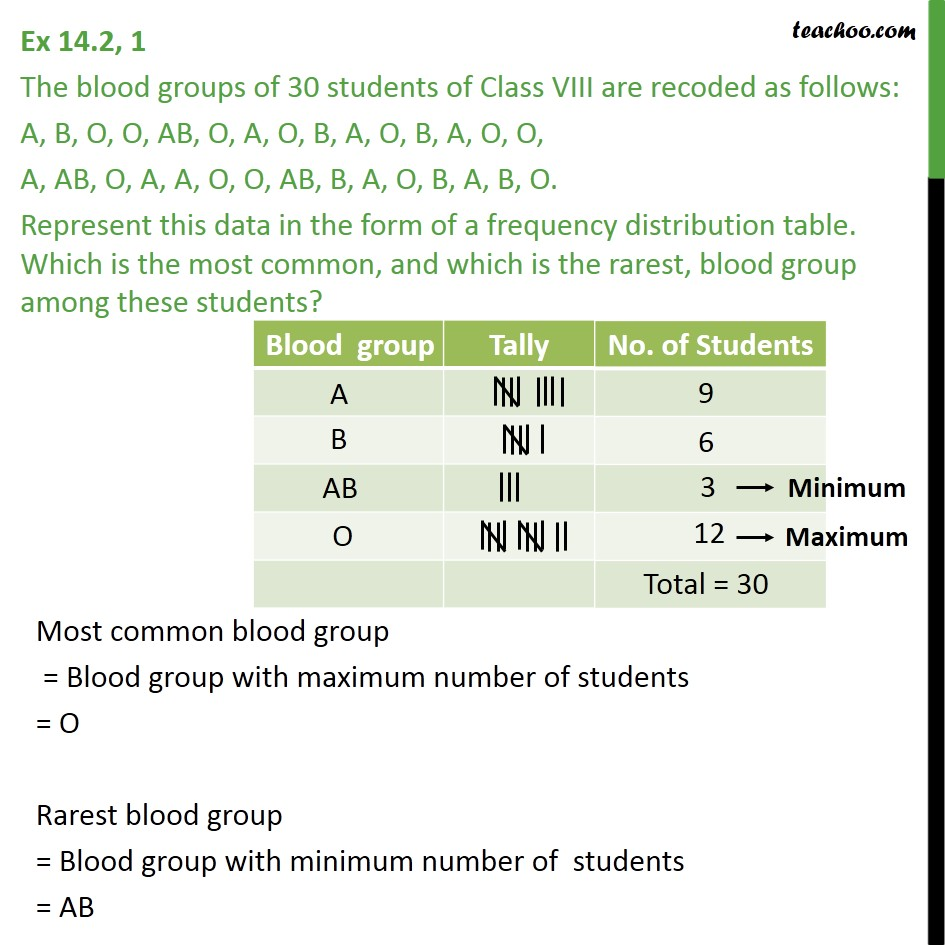Subscribe to our Youtube Channel - https://you.tube/teachoo

1. Chapter 14 Class 9 Statistics
2. Serial order wise
3. Ex 14.2

Transcript

Ex 14.2, 1 The blood groups of 30 students of Class VIII are recoded as follows: A, B, O, O, AB, O, A, O, B, A, O, B, A, O, O, A, AB, O, A, A, O, O, AB, B, A, O, B, A, B, O. Represent this data in the form of a frequency distribution table. Which is the most common, and which is the rarest, blood group among these students?

Ex 14.2

Chapter 14 Class 9 Statistics
Serial order wise